# comm.OFDMModulator

Modulate using OFDM method

## Description

The `comm.OFDMModulator` System object™ modulates a frequency domain signal by using the orthogonal frequency division multiplexing (OFDM) method. For more information, see Orthogonal Frequency Division Multiplexing. The output is a baseband representation of the OFDM-modulated signal.

To modulate a signal using OFDM:

1. Create the `comm.OFDMModulator` object and set its properties.

2. Call the object with arguments, as if it were a function.

## Creation

### Syntax

``ofdmMod = comm.OFDMModulator``
``ofdmMod = comm.OFDMModulator(Name=Value)``
``ofdmMod = comm.OFDMModulator(ofdmDemod)``

### Description

example

````ofdmMod = comm.OFDMModulator` creates an OFDM modulator System object.```

example

````ofdmMod = comm.OFDMModulator(Name=Value)` specifies Properties using one or more name-value arguments. For example, `comm.OFDMModulator(NumSymbols=8)` specifies eight OFDM symbols in the time-frequency grid.```

example

````ofdmMod = comm.OFDMModulator(ofdmDemod)` sets the OFDM modulator object properties based on the specified `comm.OFDMDemodulator` object.```

## Properties

expand all

Unless otherwise indicated, properties are nontunable, which means you cannot change their values after calling the object. Objects lock when you call them, and the `release` function unlocks them.

If a property is tunable, you can change its value at any time.

Number of FFT points, specified as a positive, integer scalar. The length of the FFT must be greater than or equal to 8 and is equivalent to the number of subcarriers.

Number of subcarriers allocated to the left and right guard bands, specified as a 2-by-1 integer vector. The number of left and right guard-band subcarriers, [NleftG; NrightG], must fall within [0,NFFT / 2 ⌋ − 1], where NFFT is the total number of subcarriers in the OFDM signal specified by `FFTLength`. For more information, see Subcarrier Allocation, Guard Bands, and Guard Intervals.

Option to insert a null DC subcarrier, specified as a numeric or logical `0` (`false`) or `1` (`true`). When inserted, the null DC subcarrier is at the center of the frequency band and has the index value:

• (NFFT / 2) + 1 when NFFT is even.

• (NFFT + 1) / 2 when NFFT is odd.

NFFT is the total number of subcarriers in the OFDM signal specified by `FFTLength`.

Option to input pilot subcarriers, specified as a numeric or logical `0` (`false`) or `1` (`true`). When you set this property to:

Indices of the pilot subcarrier locations, specified as a column vector, matrix, or 3D array with integer-element values in the range

`$\left[{N}_{\text{leftG}}+1,\text{\hspace{0.17em}}{N}_{\text{FFT}}/2\right]\cup \left[{N}_{\text{FFT}}/2+2,\text{\hspace{0.17em}}{N}_{\text{FFT}}-{N}_{\text{rightG}}\right],$`

where NFFT is the total number of subcarriers specified by `FFTLength`, and NleftG and NrightG are the left and right guard bands specified by `NumGuardBandCarriers`.

You can assign the NPilot pilot carrier indices to the same or different NSym subcarriers for each symbol, and across NT transmit antennas.

• When the pilot indices are the same for every symbol and transmit antenna, the property has dimensions of NPilot-by-1.

• When the pilot indices vary across symbols, the property has dimensions of NPilot-by-NSym.

• If the `pilot` input has only one symbol but the object configuration assigns multiple transmit antennas, the property has dimensions of NPilot-by-1-by-NT.

• If the indices vary across the number of symbols and transmit antennas, the property has dimensions of NPilot-by-NSym-by-NT.

Tip

To minimize interference between transmissions across more than one transmit antenna, the pilot indices per symbol must be mutually distinct across the antennas.

#### Dependencies

This property applies when you set `PilotInputPort` to `1`.

Length of the cyclic prefix for each OFDM symbol, specified as a positive, integer scalar or row vector containing `NumSymbols` elements. When you specify the cyclic prefix length as a:

• Scalar — The cyclic prefix length is the same for all symbols through all antennas.

• Row vector — The cyclic prefix length may vary across symbols but does not vary across antennas.

Option to apply raised cosine windowing between the OFDM symbols, specified as a numeric or logical `0` (`false`) or `1` (`true`).

To reduce the power of out-of-band subcarriers caused by spectral regrowth, apply windowing. For more information, see OFDM Raised Cosine Windowing.

Length of the raised cosine window, specified as a positive, integer scalar. This value must be less than or equal to the minimum cyclic prefix length specified by `CyclicPrefixLength`. For example, in a configuration of four symbols with cyclic prefix lengths 12, 14, 16, and 18, the window length must be less than or equal to 12.

#### Dependencies

To enable this property, set the `Windowing` property to `true`.

Oversampling factor, specified as a positive scalar. The oversampling factor must satisfy these constraints:

Tip

If you set the oversampling factor to an irrational number, specify the fractional value. For example, with an FFT length of `12` and an oversampling factor of `4/3`, their product is the integer `16`. However, rounding `4/3` to `1.333` when setting the oversampling factor results in a noninteger product of `15.9960`, which results in a code error.

Number of OFDM symbols in the time-frequency grid, specified as a positive, integer scalar.

Number of transmit antennas used to transmit the OFDM modulated signal, specified as a positive, integer scalar less than or equal to `64`.

## Usage

### Syntax

``Y = ofdmMod(X)``
``Y = ofdmMod(data,pilot)``

### Description

````Y = ofdmMod(X)` modulates the frequency-domain input data subcarriers using the OFDM method and returns the OFDM-modulated baseband signal.```
````Y = ofdmMod(data,pilot)` assigns the pilot signal, `pilot`, into the frequency subcarriers specified by `PilotCarrierIndices`. To enable this syntax, set the `PilotInputPort` property to `true`.```

### Input Arguments

expand all

Input baseband signal, specified as a NData-by-NSym-by-NT array.

Data Types: `double` | `single`
Complex Number Support: Yes

Pilot signal, specified as an NPilot-by-NSym-by-NT array.

Data Types: `double` | `single`
Complex Number Support: Yes

### Output Arguments

expand all

OFDM-modulated baseband signal, returned as an (NCPTotal + NFFT × NSym)-by-NT matrix of the same data type as the input signal.

## Object Functions

To use an object function, specify the System object as the first input argument. For example, to release system resources of a System object named `obj`, use this syntax:

`release(obj)`

expand all

 `info` Provide dimensioning information for OFDM modulator `showResourceMapping` Show subcarrier mapping of OFDM symbols created by OFDM modulator or demodulator System object
 `step` Run System object algorithm `release` Release resources and allow changes to System object property values and input characteristics `reset` Reset internal states of System object

## Examples

collapse all

Create and display an OFDM modulator System object™ with default property values.

`ofdmMod = comm.OFDMModulator`
```ofdmMod = comm.OFDMModulator with properties: FFTLength: 64 NumGuardBandCarriers: [2x1 double] InsertDCNull: false PilotInputPort: false CyclicPrefixLength: 16 Windowing: false OversamplingFactor: 1 NumSymbols: 1 NumTransmitAntennas: 1 ```

Modify the number of subcarriers and symbols. Verify that the number of subcarriers and the number of symbols changed. Use the `info` object function to display the input and output signal dimensions.

```ofdmMod.FFTLength = 128; ofdmMod.NumSymbols = 2```
```ofdmMod = comm.OFDMModulator with properties: FFTLength: 128 NumGuardBandCarriers: [2x1 double] InsertDCNull: false PilotInputPort: false CyclicPrefixLength: 16 Windowing: false OversamplingFactor: 1 NumSymbols: 2 NumTransmitAntennas: 1 ```
`info(ofdmMod)`
```ans = struct with fields: DataInputSize: [117 2] OutputSize: [288 1] ```

Use the `showResourceMapping` object function to show the mapping of data, pilot, and null subcarriers in the time-frequency space.

`showResourceMapping(ofdmMod)`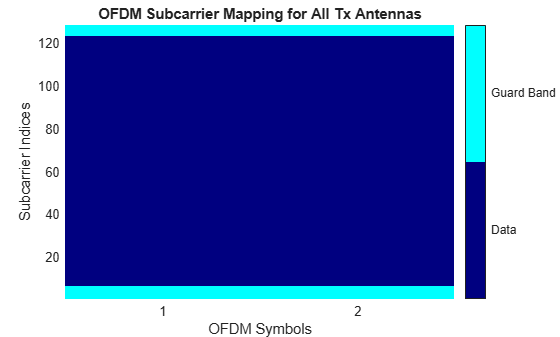Update the object to add a DC null and activate the pilot input argument. Display the configuration of the updated object. Use the `showResourceMapping` object function to show the updated resource mapping. Use the `info` object function to display the updated input and output signal dimensions. The updated input and output dimensions show the change to the object configuration. Specifically, when the object runs, pilot subcarriers must be provided.

```ofdmMod.InsertDCNull = 1; ofdmMod.PilotInputPort = 1```
```ofdmMod = comm.OFDMModulator with properties: FFTLength: 128 NumGuardBandCarriers: [2x1 double] InsertDCNull: true PilotInputPort: true PilotCarrierIndices: [4x1 double] CyclicPrefixLength: 16 Windowing: false OversamplingFactor: 1 NumSymbols: 2 NumTransmitAntennas: 1 ```
`info(ofdmMod)`
```ans = struct with fields: DataInputSize: [112 2] PilotInputSize: [4 2] OutputSize: [288 1] ```
`showResourceMapping(ofdmMod)`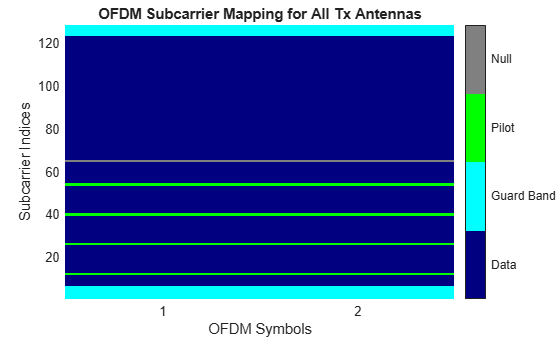Creating the modulator by using the configured demodulator ensures a matched modulator and demodulator pair.

Create an OFDM demodulator System object™ with default property values. Then, specify pilot indices for a single symbol and two transmit antennas. When you use the demodulator in the creation of the modulator, setting the `PilotCarrierIndices` property of the demodulator affects the number of transmit antennas in the OFDM modulator. The number of receive antennas in the demodulator is uncorrelated with the number of transmit antennas.

```ofdmDemod = comm.OFDMDemodulator; ofdmDemod.PilotOutputPort = true; ofdmDemod.PilotCarrierIndices = ... cat(3,[12; 26; 40; 54],[13; 27; 41; 55]);```

Use the OFDM demodulator to create the OFDM modulator.

`ofdmMod = comm.OFDMModulator(ofdmDemod);`

Display the properties of the OFDM modulator and demodulator, verifying that the applicable properties match.

`disp(ofdmMod)`
``` comm.OFDMModulator with properties: FFTLength: 64 NumGuardBandCarriers: [2x1 double] InsertDCNull: false PilotInputPort: true PilotCarrierIndices: [4x1x2 double] CyclicPrefixLength: 16 Windowing: false OversamplingFactor: 1 NumSymbols: 1 NumTransmitAntennas: 2 ```
`disp(ofdmDemod)`
``` comm.OFDMDemodulator with properties: FFTLength: 64 NumGuardBandCarriers: [2x1 double] RemoveDCCarrier: false PilotOutputPort: true PilotCarrierIndices: [4x1x2 double] CyclicPrefixLength: 16 OversamplingFactor: 1 NumSymbols: 1 NumReceiveAntennas: 1 ```

The `showResourceMapping` object function displays the time-frequency resource mapping for each transmit antenna.

Create an OFDM modulator.

`ofdmMod = comm.OFDMModulator;`

Apply the `showResourceMapping` object function.

`showResourceMapping(ofdmMod)`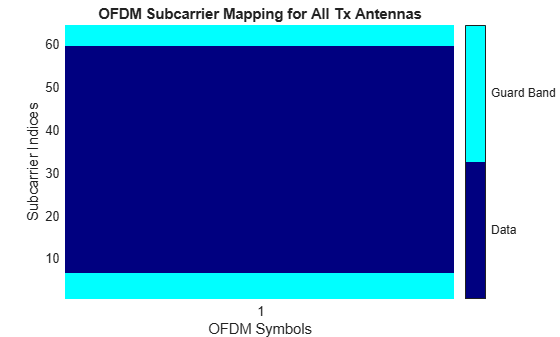Insert a DC null.

`ofdmMod.InsertDCNull = true;`

Show the resource mapping after adding the DC null.

`showResourceMapping(ofdmMod)`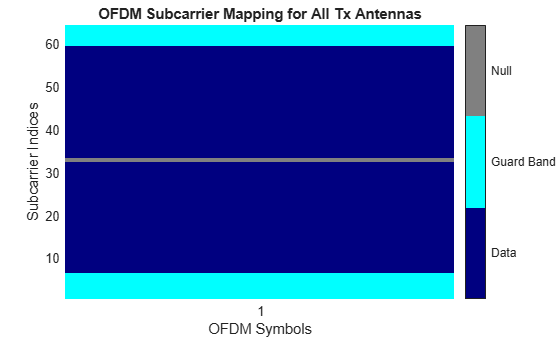Create an OFDM modulator and specify the subcarrier indices for the pilot signals. Specify the indices for each symbol and transmit antenna. When the number of transmit antennas is greater than one, set different pilot indices for each symbol between antennas.

Create an OFDM modulator object, specifying two symbols and inserting a DC null.

```ofdmMod = comm.OFDMModulator( ... FFTLength=128, ... NumSymbols=2, ... InsertDCNull=true);```

Enable the pilot input port so you can specify the pilot indices.

`ofdmMod.PilotInputPort = true;`

Specify the same pilot indices for both symbols.

`ofdmMod.PilotCarrierIndices = [12; 56; 89; 100];`

Display the placement of the pilot signals and nulls in the OFDM time-frequency grid by using the `showResourceMapping` object function.

`showResourceMapping(ofdmMod)`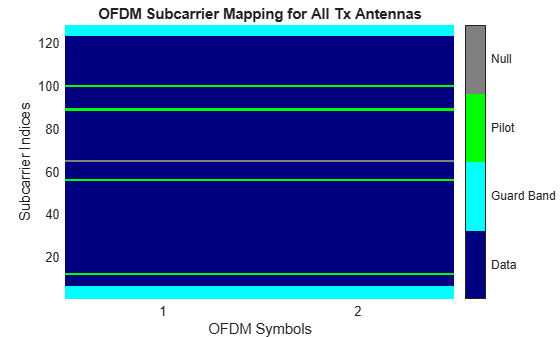Specify different indices for the second symbol by concatenating a second column of pilot indices to the `PilotCarrierIndices` property.

```ofdmMod.PilotCarrierIndices = ... cat(2,ofdmMod.PilotCarrierIndices,[17; 61; 94; 105]);```

Verify that the pilot subcarrier indices differ between the two symbols.

`showResourceMapping(ofdmMod)`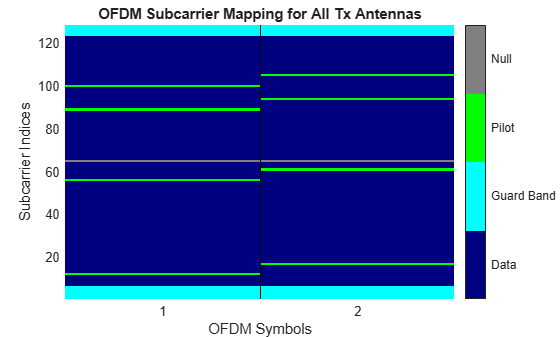Increase the number of transmit antennas to two.

`ofdmMod.NumTransmitAntennas = 2;`

Specify the pilot indices for each of the two transmit antennas. To provide indices for multiple antennas while minimizing interference among the antennas, specify the `PilotCarrierIndices` property as a 3D array so that the indices for each symbol differ among antennas.

```ofdmMod.PilotCarrierIndices = ... cat(3,[20; 50; 70; 110],[15; 60; 75; 105]);```

Display the resource mapping for the two transmit antennas. The gray lines denote the insertion of custom nulls. To minimize interference among the pilot symbols from different antennas, the object creates nulls.

`showResourceMapping(ofdmMod)`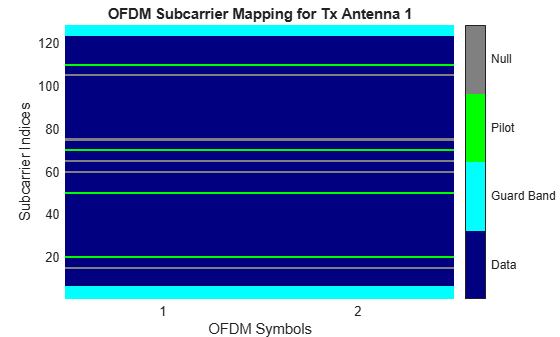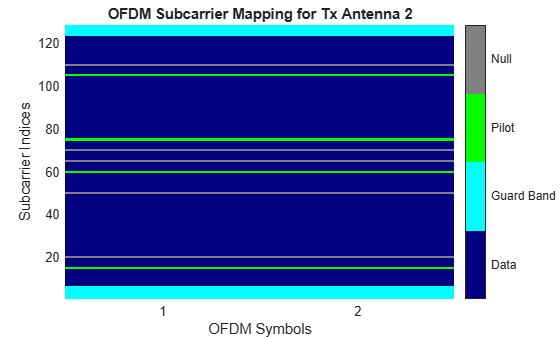Specify the length of the cyclic prefix for each OFDM symbol.

Create an OFDM modulator, specifying five symbols, four left and three right guard-band subcarriers, and the cyclic prefix length for each OFDM symbol.

```ofdmMod = comm.OFDMModulator( ... NumGuardBandCarriers=[4;3], ... NumSymbols=5, ... CyclicPrefixLength=[12 10 14 11 13]);```

Display the properties of the OFDM modulator to confirm that the configured cyclic prefix length changes across the symbols.

`disp(ofdmMod)`
``` comm.OFDMModulator with properties: FFTLength: 64 NumGuardBandCarriers: [2x1 double] InsertDCNull: false PilotInputPort: false CyclicPrefixLength: [12 10 14 11 13] Windowing: false OversamplingFactor: 1 NumSymbols: 5 NumTransmitAntennas: 1 ```

Get the OFDM modulator data dimensions by using the `info` object function.

Create an OFDM modulator object with pilot indices, a DC null, and two transmit antennas.

```ofdmMod = comm.OFDMModulator( ... NumGuardBandCarriers=[4; 3], ... PilotInputPort=true, ... PilotCarrierIndices=cat(3,[12; 26; 40; 54],[11; 25; 39; 53]), ... InsertDCNull=true, ... NumTransmitAntennas=2);```

Use the `info` object function to get the modulator input data, pilot input data, and output data sizes.

`info(ofdmMod)`
```ans = struct with fields: DataInputSize: [48 1 2] PilotInputSize: [4 1 2] OutputSize: [80 2] ```

Generate OFDM modulated symbols for use in link-level simulations.

Construct an OFDM modulator with an inserted DC null, seven guard-band subcarriers, and two symbols with different pilot indices for each symbol.

```ofdmMod = comm.OFDMModulator( ... NumGuardBandCarriers=[4;3], ... PilotInputPort=true, ... PilotCarrierIndices=[12 11; 26 27; 40 39; 54 55], ... NumSymbols=2, ... InsertDCNull=true);```

Determine input data, pilot, and output data dimensions.

`modDim = info(ofdmMod);`

Generate random data symbols for the OFDM modulator. The structure variable, `modDim`, determines the number of data symbols.

```dataIn = complex( ... randn(modDim.DataInputSize),randn(modDim.DataInputSize));```

Create a pilot signal that has the correct dimensions.

```pilotIn = complex( ... rand(modDim.PilotInputSize),rand(modDim.PilotInputSize));```

Apply OFDM modulation to the data and pilot signals.

`modData = ofdmMod(dataIn,pilotIn);`

Use the OFDM modulator object to create the corresponding OFDM demodulator.

`ofdmDemod = comm.OFDMDemodulator(ofdmMod);`

Demodulate the OFDM signal and output the data and pilot signals.

`[dataOut, pilotOut] = ofdmDemod(modData);`

Verify that, within a tight tolerance, the input data and pilot symbols match the output data and pilot symbols.

```isSame = (max(abs([dataIn(:) - dataOut(:); ... pilotIn(:) - pilotOut(:)])) < 1e-10)```
```isSame = logical 1 ```

Filter an OFDM modulated signal with data and pilot inputs and outputs generated at four times the sample rate through a single-input single-output (SISO) channel. Demodulate the channel-filtered signal and compare to the original data.

Create an OFDM demodulator object that has three symbols and different pilot subcarrier indices and cyclic prefix lengths for each symbol.

```ofdmDemod = comm.OFDMDemodulator( ... NumGuardBandCarriers=[9;8], ... RemoveDCCarrier=true, ... PilotOutputPort=true, ... PilotCarrierIndices=[[12;26;40;54],[14;28;38;52],[12;26;40;54]], ... CyclicPrefixLength=[16 32 16], ... OversamplingFactor=4, ... NumSymbols=3);```

Create an OFDM modulator System object from the OFDM demodulator object, `ofdmDemod`.

`ofdmMod = comm.OFDMModulator(ofdmDemod);`

Show the configured subcarrier resource mapping for data, pilot, guard band and null signals by using the `showResourceMapping` object function. Obtain the input and output dimension information by using the `info` object function.

`showResourceMapping(ofdmDemod);`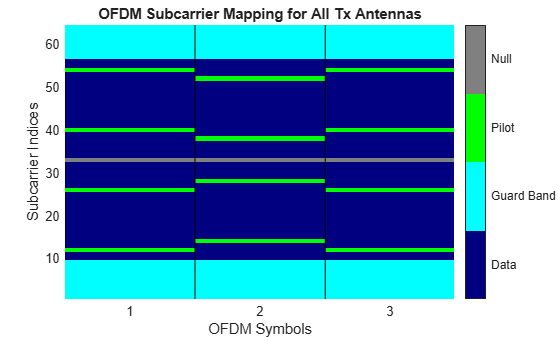`modDim = info(ofdmMod);`

Create random data and pilot inputs and apply QAM modulation.

```M = 16; xd = randi([0 M-1],modDim.DataInputSize); dataIn = qammod(xd,M,UnitAveragePower=true); xp = randi([0 M-1],modDim.PilotInputSize); pilotIn = qammod(xp,M,UnitAveragePower=true);```

Apply OFDM modulation to the data and pilot QAM signals. Filter the signal through an AWGN channel. To recover the data and pilot symbols, apply OFDM demodulation and then QAM-demodulation.

```modOut = ofdmMod(dataIn,pilotIn); chanOut = awgn(modOut,20,"measured"); [dataOut, pilotOut] = ofdmDemod(chanOut); yd = qamdemod(dataOut,M,UnitAveragePower=true); yp = qamdemod(pilotOut,M,UnitAveragePower=true);```

Verify that the data and pilots are unchanged through this process.

`dataSame = isequal(xd,yd)`
```dataSame = logical 1 ```
`pilotSame = isequal(xp,yp)`
```pilotSame = logical 1 ```

## Algorithms

expand all

 Dahlman, E., S. Parkvall, and J. Skold. 4G LTE/LTE-Advanced for Mobile Broadband.London: Elsevier Ltd., 2011.

 Andrews, J. G., A. Ghosh, and R. Muhamed, Fundamentals of WiMAX, Upper Saddle River, NJ: Prentice Hall, 2007.

 Agilent Technologies, Inc., "OFDM Raised Cosine Windowing", https://rfmw.em.keysight.com/wireless/helpfiles/n7617a/ofdm_raised_cosine_windowing.htm.

 Montreuil, L., R. Prodan, and T. Kolze. "OFDM TX Symbol Shaping 802.3bn", https://www.ieee802.org/3/bn/public/jan13/montreuil_01a_0113.pdf. Broadcom, 2013.

 IEEE Standard 802.16-2017. "Part 16: Air Interface for Broadband Wireless Access Systems." March 2018.